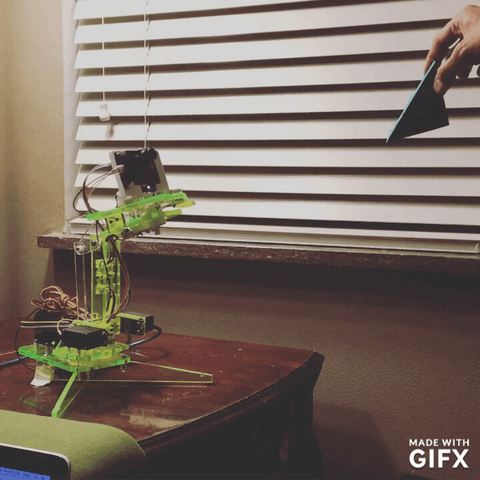# mimicArmPixyControlIn this experiment the mimicArm programmable robot arm follows a block of a specific color using the PixyCam 5.  The experiment requires the PixyCam 5 and a mount for mimicArm. You will also need to download the PixyCam Library. In addition, this example uses functions, and makes a complex behavior simple. For information on hooking up the PixyCam and using the PixyCam software see here.

The command "getblocks()" tells you how many blocks of a given color the PixyCam can see. For simplicity we only look for the first block, so all commands after that use blocks. The code simple finds the block, and identifies if it is to the left, right, top, or bottom of the frame. The robot is then moved to center the block in the frame.

## Commands Used:

pixy.init()

pixy.getblocks()

pixy.blocks[block].x

pixy.blocks[block].y

robotActivate()

robotMove(channel, position)

robotJog(channel, position)

## Cut and paste code:

#include <SPI.h>
#include <Pixy.h>
#include <robot.h>

// This is the main Pixy object
Pixy pixy;

void setup()
{
Serial.begin(9600);
Serial.print("Starting...\n");
delay(2000);
robotActivate();
robotHome();
pixy.init();

}

void loop()
{
robotMode(arduino);
static int i = 0;
int j;
uint16_t blocks;
char buf;
//robotMode(arduino);

// grab blocks!
blocks = pixy.getBlocks();
//Serial.println(blocks);
//Serial.println(distance);

// If there are detect blocks, print them!
if (blocks)
{
//Serial.println("got here");
//Serial.print("x is \t");
//Serial.print(pixy.blocks.x);
//Serial.print("\ty is \t");
//Serial.println(pixy.blocks.y);
//if (distance > 150) {  //this needs to be inside if(blocks) so it doens't jump at shadows
//  Serial.print("satisfied ");
//  robotJog(2, -1);
//}

if (pixy.blocks.x < 150) robotJog(1, -1);
else if (pixy.blocks.x > 170) robotJog(1, 1);
if (pixy.blocks.y < 90) robotJog(3, 1);
else if (pixy.blocks.y > 110) robotJog(3, -1);
}
Serial.print("position is ");
Serial.println(robotPosition(2));
//if ((distance < 150) && (robotPosition(2) <= 127)) robotJog(2, 1);  //this needs to be outside if(blocks) so it moves back when block removed

//else robotHome();
delay(20);
}

void robotHome() {
robotMove(1, 127);
robotMove(3, 127);
robotMove(2, 127);
}